Courses

# BJT As An Amplifier - 2

## 15 Questions MCQ Test Topicwise Question Bank for GATE Electrical Engineering | BJT As An Amplifier - 2

Description
This mock test of BJT As An Amplifier - 2 for Electrical Engineering (EE) helps you for every Electrical Engineering (EE) entrance exam. This contains 15 Multiple Choice Questions for Electrical Engineering (EE) BJT As An Amplifier - 2 (mcq) to study with solutions a complete question bank. The solved questions answers in this BJT As An Amplifier - 2 quiz give you a good mix of easy questions and tough questions. Electrical Engineering (EE) students definitely take this BJT As An Amplifier - 2 exercise for a better result in the exam. You can find other BJT As An Amplifier - 2 extra questions, long questions & short questions for Electrical Engineering (EE) on EduRev as well by searching above.
QUESTION: 1

### The value of input impedance and voltage gain of the common base circuit shown below are respectively (Take VBE = 0.6 V)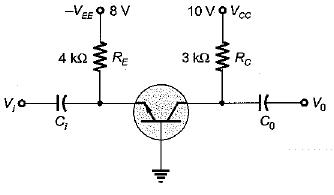Solution:

Using AC equivalent circuit for the common base configuration, we can find that,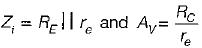Now,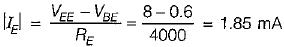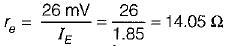∴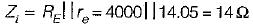and AV = voltage gain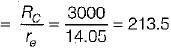QUESTION: 2

### The circuit shown in figure is biased such that VCE2 = 0 when VS = 0. Neglecting the base current of both transistors and forward bias across VBE  for both transistors to be 0.7, the value of RE would be equal to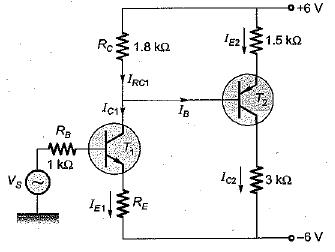Solution:

Applying KVL, we have: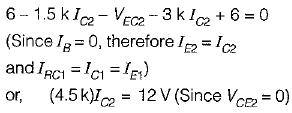or,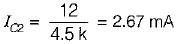Also,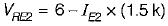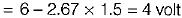∴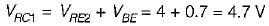∴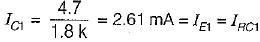When, Vi = 0,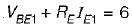or,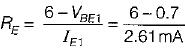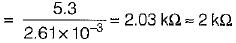QUESTION: 3

### A cascade stage is shown in figure below. If the transconductance of T1 and T2 are gm1 = ic1 / Vbe1 and gm2 = ic2 / Vbe2, the overall transconductance gm = ic2 / Vbe1 is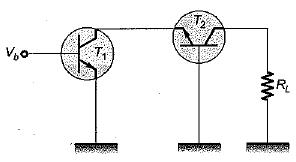Solution: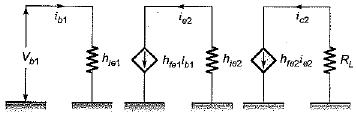Here,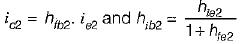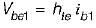Also,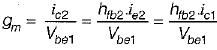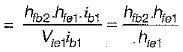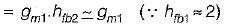Hence, gm = gm1

QUESTION: 4

An emitter follower with β = 100 is biased at IC - 0.25 mA. The voltage source connected at its input has its internal resistance of 2 kΩ What is the value of RE such that it produces the output resistance of 110 Ω (Take VT = 25 mV)

Solution: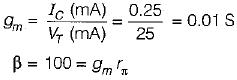or,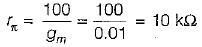Now,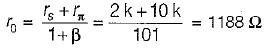Desired effective output resistance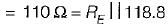or,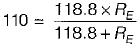or,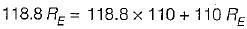or,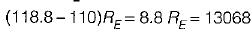or,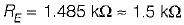QUESTION: 5

Two stages of BJT amplifiers are cascaded by RC coupling. The voltage gain of the first stage is 20 and that of the second stage is 30. The overall gain of the coupled amplifier is

Solution:

The voltage gain of a multi-stage amplifier is equal to the product of the gains of the individual stages.

QUESTION: 6

The main characteristics of a Darlington amplifier are

Solution:
QUESTION: 7

Which of the following parameters is used for distinguishing between a small signal and a large-signal amplifier?

Solution:
QUESTION: 8

Consider the following statements:
1. The coupling capacitor mainly affect the lower cutoff frequency.
2. The phase reversal between output and input takes place only for voltage wave and not for current waves, in a transistor amplifier in CE mode.
3. The DC collector current in a transistor circuit is limited by the junction capacitance.
4. Negative DC feedback through RE is responsible for the stabilization of the operating point in a potential divider bias circuit.
​Of these statement:

Solution:
QUESTION: 9

A transistor has hfe = 100, hte = 5.2 kΩ, and rbb, = 0. At room temperature V= 26 mV. The collector current |IC| will be

Solution:

hje= rbb' + rπ
Here, rbb' = 0
Therefore,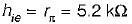Now,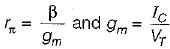∴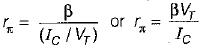∴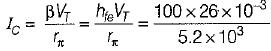= 0.5 mA

QUESTION: 10

A transistor is used in common emitter mode in an amplifier circuit. When a signal of 30 mV is added to the base emitter voltage, the base current changes by 30 μA and collector current by 3 mA. The load resistance is 5 kΩ. The value of transconductance and voltage gain is given by

Solution: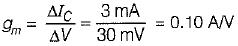and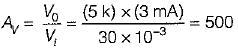QUESTION: 11

The transistor in the amplifier shown has the following parameters:
hfe = 100, hie = 2 kΩ, hre = 0, hoe = 0.05 m Mho.
'C’ is very large. The output impedance is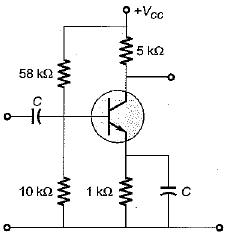Solution: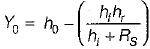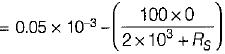or,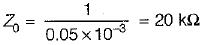QUESTION: 12

If RS is the source resistance, the output resistance of an emitter-follower using the simplified hybrid model would be

Solution:

If RS is the source resistance, the output resistance of an emitter-follower using the simplified hybrid model would be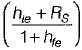QUESTION: 13

In the CE amplifier with RL = 4000 Ω, given that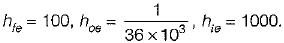The current gain |Ai| is

Solution:

Current gain,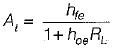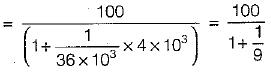or,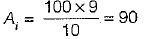QUESTION: 14

Assertion (A): On extending the hybrid model for two-port network to a transistor it is assumed that the signal excursion about the Q-point is small.
Reason (R): Small signal operation is that in which the AC input signal voltages and currents are in order of ±10% of Q-point voltage and currents.

Solution:

Both assertion and reason are correct statements.
The correct reason for assuming small signal excursion about the Q-point is to keep the transistor parameters constant over the signal excursion.

QUESTION: 15

For a common-collector configuration of an n-p-n transistors, the hybrid parameters are given as:
hic = hie, hrc = - 1, hfc = - (1 + hfe) and hoc = hoe.
Match List-I (Hybrid parameters of CB configuration) with List-II (Values) and select the correct answer using the codes given below the lists: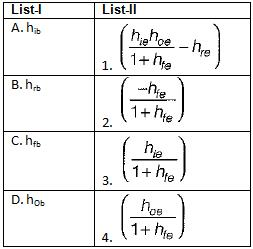Codes: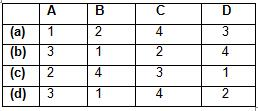Solution: